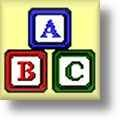#Kindergarten Worksheets

## Dynamically Created Kindergarten Worksheets

Here is a graphic preview for all of the kindergarten worksheets. You can select different variables to customize these kindergarten worksheets for your needs. The kindergarten worksheets are randomly created and will never repeat so you have an endless supply of quality kindergarten worksheets to use in the classroom or at home.

## Detailed Description for All Kindergarten Worksheets

Number Recognition
These kindergarten worksheets will produce number recognition worksheets for all numbers between 0 and 20. These kindergarten worksheets are great classroom aids.

Shape Recognition
These kindergarten worksheets will produce shape recognition worksheets for 13 different shapes. These shapes include circles, squares, rectangles, diamonds, trapezoids, hexagons, pentagons, stars, hearts, and many more that may be used with your kindergarten class.

Color the Shapes
These kindergarten worksheets will produce a worksheet of different shapes and the children will color the shapes based on the selections you make. These kindergarten worksheets are great classroom activities.

Writing Numbers
These kindergarten worksheets will produce practice worksheets for writing numbers between 0 and 9. These kindergarten worksheets are practice worksheets.

Identifying Numbers
These kindergarten worksheets will produce ten numbers in a select range for the students to identify. The number may be listed with or without duplicates.

Place Values Practice
These kindergarten worksheets will make Place Value Problems that can be colored, cut and pasted to teach place values. These kindergarten worksheets are great classroom activities.

Biggest and Smallest
These kindergarten worksheets will produce four problems where the children will select which picture is either the biggest or smallest. These kindergarten worksheets are great practice material.

Greater Than Less Than
These kindergarten worksheets will produce sixteen problems where the children will cut out alligators for greater than and less than and paste for the correct answer. These kindergarten worksheets are great classroom activities.

Connect the Dots
These kindergarten worksheets will produce connect the dot pictures for the children to practice counting and then coloring the pictures. These kindergarten worksheets are great classroom activities.

Matching Numbers to Objects
These kindergarten worksheets will produce problems where it asks the children to match the number with the correct group of objects. These kindergarten worksheets are great classroom activities.

Matching Numbers to Their Names
These kindergarten worksheets will produce problems where it asks the children to match the numbers with the word for that number. These kindergarten worksheets are practice worksheets.

Matching Shapes to Their Names
These kindergarten worksheets will produce problems where it asks the children to match the shape with the word for that shape. These kindergarten worksheets are practice worksheets.

Matching US Currency to Their Names
These kindergarten worksheets will produce problems where it asks the children to match US Currency with the word for that currency. These kindergarten worksheets are practice worksheets.

Comparing Objects and Numbers
These kindergarten worksheets will produce problems where it asks the children to circle the group of objects that match the given number. These kindergarten worksheets are practice worksheets.

Count How Many
These kindergarten worksheets will produce problems where it asks the children to count how many objects are in the group. These kindergarten worksheets will generate 6 problems per worksheet.

Count the Number of Tally Marks
These kindergarten worksheets will produce ten problems where the children will count the number of tally marks. These kindergarten worksheets will generate 10 problems per worksheet.

Counting Coins
These kindergarten worksheets will produce problems with randomly generated coins. You have the option to select any combination of pennies, nickels, dimes, and quarters for each new kindergarten worksheets.

Custom Counting Coins
These kindergarten worksheets will give you the options to select the exact combination of different coins that will be used for each problem. Depending on coin selection you can generate up to 9 problems.

Skip Counting
These kindergarten worksheets will produce skip counting problems where it asks the children to complete the series. You may select the skip to be between the number range of 2 and 12. These kindergarten worksheets will generate 10 problems per worksheet.

Custom Skip Counting
These kindergarten worksheets will produce custom Skip Counting problems with ten numbers in the series. You may select the starting and skip numbers individually for each problem. This kindergarten worksheet will generate 10 problems per page.

Fill in the Missing Numbers
These kindergarten worksheets will produce worksheet problems where it asks the children to fill in the missing numbers in a series. These kindergarten worksheets will generate 6 problems per worksheet.

Complete the Series
These kindergarten worksheets will produce problems where it asks the children to complete the missing numbers in a series. These kindergarten worksheets will generate 10 problems per worksheet.

Complete the Pattern
These kindergarten worksheets will produce a simple series of patterns. The children will complete the next object in the series. You may select the series to be shapes, colors or shapes and colors. These kindergarten worksheets will generate 10 problems per worksheet.

These kindergarten worksheets will produce adding shape problems where the children will add the two different groups of shapes for the total number of shapes. These kindergarten worksheets will generate 8 problems per worksheet.

Subtracting Shapes
These kindergarten worksheets will produce subtracting shape problem where the children will subtract the two different groups of shapes for the total number of shapes left. These kindergarten worksheets will generate 8 problems per worksheet.